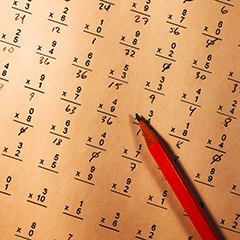# Maths and stat skills

## Maths#### Essential Numeracy (UON Prep course)

This online course aims to remind students of the fundamental rules of arithmetic which underpin all higher levels of mathematics.
Topics: order of operations, whole number arithmetic, fractions, decimals, percentages.

#### Maths Skills for Nursing

Maths skills underpinning nursing calculations.
Topics include: multiplication, long division, decimals, fractions and using formulas.

#### Maths Tuneup

Packed full of explanations, examples and practice questions of some of the fundamental maths concepts. Introductions to algebra, problem solving and calculus.

#### Algebraic Concepts for Accounting and Finance

This Blackboard learning module covers algebraic skills that are often assumed knowledge in accounting and finance courses.
Topics include: solving and rearranging equations, logarithms.

#### How to learn maths

The Getting Ahead in Mathematics (GAIM) series of websites provides instructional videos and information on a range of topic areas:

## Stats#### Stats Tuneup

Provides an overview of key statistical concepts and methods. Includes videos, practice questions and worked solutions.

#### Statistical Support Service

Advice on statistical methods to help university students and staff engaged in research.